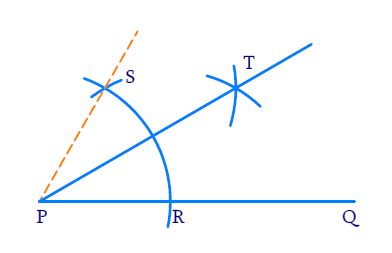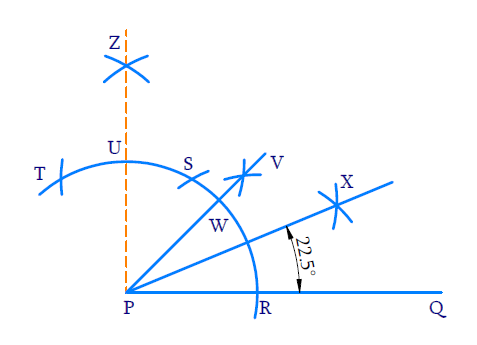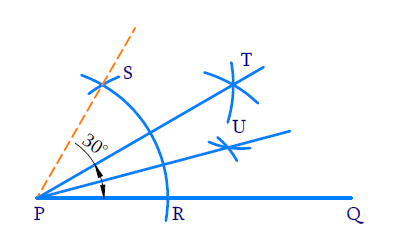# Ex.11.1 Q3 Constructions Solution - NCERT Maths Class 9

Go back to  'Ex.11.1'

## Question

Construct the angles of the following measurements:

(i) $$30^{\circ}$$

(ii) $$22 \frac{1}{2}^{\circ}$$

(iii) $$15^{\circ}$$

Video Solution
Constructions
Ex 11.1 | Question 3

## Text Solution

$${\rm{(i)}}\;\;30^{\circ}$$

Reasoning:

We need to construct an angle of $$60$$ degrees and then bisect it to get an angle measuring $$30^\circ$$

Steps of Construction:(i) Draw ray $$PQ$$.

(ii) To construct an angle of $$60^{\circ}$$ .

With $$P$$ as centre and any radius, draw a wide arc to intersect $$PQ$$ at $$R$$. With $$R$$ as centre and same radius draw an arc to intersect the initial arc at $$S$$. $$\angle {SPR}=60^{\circ}$$

(iii) To bisect $$\angle {SPR}$$

With $$R$$ and $$S$$ as centres and same radius draw two arcs to intersect at $$T$$. Join $$P$$ and $$T$$ i.e. $$PT$$ is the angle bisector. Hence,

\begin{align}\angle {TPR}=\frac{1}{2} \angle {SPR}=30^{\circ}\end{align}

$${\rm{(ii)}}\;\;22 \frac{1}{2}^{\circ}$$

Reasoning:

We need to construct two adjacent angles of and bisect the second one to get a angle. This has to be bisected again to get a $$45^\circ$$ angle. The $$45^\circ$$ angle has to be further bisected to get \begin{align}22 \frac{1}{2}^{\circ}\end{align} angle.

\begin{align} 22 \frac{1}{2}^{\circ} &=\frac{45^{\circ}}{2} \\ 45^{\circ} &=\frac{90^{\circ}}{2}=\frac{30^{\circ}+60^{\circ}}{2} \end{align}

Steps of Construction:(i) Draw ray $$PQ$$

(ii) To construct an angle of $$60^{\circ}$$

With $$P$$ as center and any radius draw a wide arc to intersect $$PQ$$ at $$R$$. With $$R$$ as center and same radius draw an arc to intersect the initial arc at $$S$$. $$\angle {SPR}=60^{\circ}$$

(iii)To construct adjacent angle of $$60^{\circ}$$ .

With $$S$$ as the center and same radius as before, draw an arc to intersect the initial arc at $$T$$ $$\angle {TPS}=60^{\circ}$$ .

(iv)To bisect $$\angle {TPS}$$

With $$T$$ and $$S$$ as centers and same radius as before, draw arcs to intersect each other at $$Z$$ Join $$P$$ and $$Z$$ $$\angle {ZPQ}=90^{\circ}$$

(v) To bisect $$\angle {ZPQ}$$

With $$R$$ and $$U$$ as centers and radius than half of $$RU$$, draw arcs to intersect each other at $$V$$. Join $$P$$ and $$V$$. $$\angle {VPQ}=45^{0}$$

(vi) To bisect $$\angle {VPQ}=45^{0}$$

With $$W$$ and $$R$$ as centers and radius greater than half of $$WR$$, draw arcs to intersect each other at $$X$$. Join $$P$$ and $$X$$. $$PX$$ bisects $$\angle {VPQ}$$

Hence,

\begin{align} \angle {XPQ} &=\frac{1}{2} \angle {WPQ} \\ &=\frac{1}{2} \times 45^{0} \\ &=22 \frac{1}{2} \end{align}

$${\rm{(iii)}}\;\;15^{\circ}$$

Reasoning:

We need to construct an angle of 60 degrees and then bisect it to get an angle measuring $$30^\circ$$. This has to be bisected again to get a $$15^\circ$$ angle.

\begin{align}15^{0}=\frac{30^{\circ}}{2}=\frac{\frac{60^{0}}{2}}{2}\end{align}

Steps of Construction:(i) Draw ray $$PQ$$.

(ii) To construct an angle of $$60^{\circ}$$ .

With $$P$$ as center and any radius draw a wide arc to intersect $$PQ$$ at $$R$$. With $$R$$ as center and same radius draw an arc to intersect the initial arc at $$S$$. $$\angle {SPR}=60^{\circ}$$

(iii) Bisect $$\angle {SPR}$$ . With $$R$$ and $$S$$ as centers and radius greater than half of $$RS$$ draw arcs to intersect each other at $$T$$. Join $$P$$ and $$T$$ i.e. $$PT$$ is the angle bisector of $$\angle {SPR}$$ .

\begin{align} \angle {TPQ} &=\frac{1}{2} \angle{SPR} \\ &=\frac{1}{2} \times 60^{\circ} \\ &=30^{\circ} \end{align}

(iv)To bisect $$\angle {TPQ}$$

With $$R$$ and $$W$$ as centers and radius greater than half of $$RT$$, draw arcs to intersect each other at $$U$$ Join $$P$$ and $$U$$. $$PU$$ is the angle bisector of $$\angle {TPQ}$$ .

\begin{align}\angle {UPQ}=\frac{1}{2} \angle {TPQ}=15^{\circ}\end{align}

Learn from the best math teachers and top your exams

• Live one on one classroom and doubt clearing
• Practice worksheets in and after class for conceptual clarity
• Personalized curriculum to keep up with school